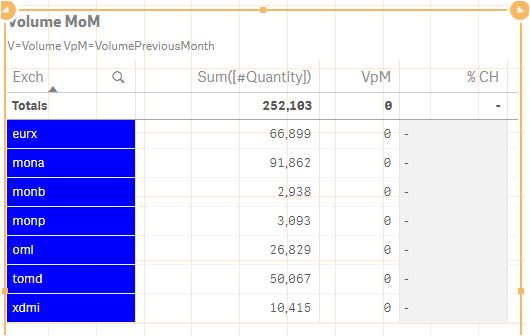# New to Qlik Sense

Discussion board where members can get started with Qlik Sense.

HighlightedNot applicable

## Table formula for previous month data

Hi,

Within this overview I have selected one month of data. In the column Sum([#quantity]) the volumes per sublevel are depicted.

Now, in the next column I want the volume of the previous month. Currently this is set at:

sum({<Month=, Date={">=\$(=monthstart(max(Date),-1))<=\$(=monthend(max(Date),-1))"}>} [#Quantity])

However, it does not work as you can see. Any idea how to resolve this?

The field is Month, and the data is Sum([#quantity])Tags (4)
13 RepliesPartner

## Re: Table formula for previous month data

try this expressionMVP

## Re: Table formula for previous month data

What is your date field format? May be try like this:

Sum({<Month =, Date = {">=\$(=Date(MonthStart(Max(Date), -1), 'DateFieldFormatHere'))<=\$(=Date(MonthEnd(Max(Date), -1), 'DateFieldFormatHere'))"}>} [#Quantity])Not applicable

## Re: Table formula for previous month data

Liron, thanks for helping out...tried both with DateFieldFormatHere= 'Date'

Sum({<Month =, Date = {">=\$(=Date(MonthStart(Max(Date), -1), 'Date'))<=\$(=Date(MonthEnd(Max(Date), -1), 'Date'))"}>} [#Quantity])

your first option did not work either...MVP

## Re: Table formula for previous month data

Date is not a format. Format would be something like this DD/MM/YYYY or DD-MM-YYYY or MM/DD/YYYY and so on.....

Assuming it is DD/MM/YYYY, you can try this

Sum({<Month =, Date = {">=\$(=Date(MonthStart(Max(Date), -1), 'DD/MM/YYYY'))<=\$(=Date(MonthEnd(Max(Date), -1), 'DD/MM/YYYY'))"}>} [#Quantity])Not applicable

## Re: Table formula for previous month data

Sunny

seems that the malfunction is in the Month field....in this field Month is January, February etc....in another app I used I had the field #month , month being 1,2, 3 etc..

how would that change things??MVP

## Re: Table formula for previous month data

The expression is not even using Month field to cause any issue. If you still wish to change Month you can try this in the script:

Num(Month(Date#(Month, 'MMMM'))) as Month

Coming back to your current issue, would you be able to share a filter box to show the format for Date field?Not applicable

## Re: Table formula for previous month data

Month is actually "Monthname'', will try to adjust that ...Not applicable

## Re: Table formula for previous month data

so the Date field is Date and the Month field is Monthname

Date format is DD/MM/YYYY

any idea what the formula would look like (e.g. October -1 obviously goes wrong..)?

thanks againMVP

## Re: Table formula for previous month data

Can you check what do you get when you use this in a KPI object

='>=' & Date(MonthStart(Max(Date), -1), 'DD/MM/YYYY') & '<=' & Date(MonthEnd(Max(Date), -1), 'DD/MM/YYYY')

Does the above give you the right date range in the right format? If it does, then this should work for you

Sum({<Month =, Date = {"\$(='>=' & Date(MonthStart(Max(Date), -1), 'DD/MM/YYYY') & '<=' & Date(MonthEnd(Max(Date), -1), 'DD/MM/YYYY'))"}>} [#Quantity])

Just added the above KPI object expression within the dollar sign expansion